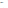# Use the Substitution Method on the Systems of Equations

The substitution method is one of the ways to solve a system of linear equations. Using this method, you isolate the variables and substitute one of them to solve for the other. These are worksheets you can use to practice the method.

01
of 06

## Substitution Method Worksheet 1 of 6

Answers are on the second page of the PDF.

y = −3x
y = x − 8

y = 3 x
y = −8x

y = −2x
y =‐4x + 10

y = −7x
y =−4x − 12

y = 3 x
y =2x − 7

y = 2 x + 3
y = 3

y = 6x + 22
y = −8

y =2 x – 5
y =x

y = 4x + 10
y = −6

y = 8
y =−2 x + 22

y = 4
y =4 x − 24

y = −6 x
y =−3x

02
of 06

## Substitution Method Worksheet 2 of 6

Answers are on the second page of the PDF.

y = −7x
y = −7

y = −6
y = −7 x + 1

y = −4
y =−6 x − 4

y = 3x − 3
y = −3

y = 3x − 1
y = −1

y = 0
y = 4x

y =−4x − 1
y = 3

y = 2
y =5x + 7

y = 6 x
y =−3x – 9

y = 2x
y =−2x + 24

y = −5 x
y =6x + 11

y = 2
y = −6 x − 22

03
of 06

## Substitution Method Worksheet 3 of 6Substitution Method Worksheet. D. Russell Print the worksheet to solve the linear equations using the substitution method.

Answers are on the second page of the PDF.

y =3x + 1
y = 7

y = −2x
y =8x – 10

y =x − 12
y = −2x

y =5
x − 6y = 7x

y = −2x
y =2x – 20

y =−4x + 16
y = −2x

y =−2x − 6
y = −8x

y =−5x + 5
y = −6x

y =3x + 14
y = 5

y = 2x
y =6x + 8

y = 5x
y =8x – 24

y =7x + 24
y = 3

04
of 06

## Substitution Method Worksheet 4 of 6

Answers are on the second page of the PDF.

y = −7x
y =−x – 4

y = −4
y =−2x – 2

y =−3x − 12
y = −6

y = 8
y =x + 8

y = 3
y =−3x – 21

y = −6
y =−7x – 6

y =−8x − 8
y = −8

y = 3
y =x – 2

y =2x − 1
y = −3

y = 3x − 23
y = −2

11.) y = −6x
y =−7x – 6

12.) y = −4x
y =−5x − 5

05
of 06

## Substitution Method Worksheet 5 of 6Substitution Method Worksheet. D. Russell Print the worksheet to solve the linear equations using the substitution method.

Answers are on the second page of the PDF.

y = 5
y =2x – 9

y =5x − 16
y = 4

y = −4x + 24
y = −7x

y = x + 3
y = 8

y = −8
y =−7x + 20

y = −7x + 22
y = −6

y = −5
y =−x + 19

y =4x + 11
y = 3

y =−6x + 6
y = −5x

y = −8
y =5x + 22

y =−2x − 3
y = −5x

y =−7x − 12
y = −4

06
of 06

## Substitution Method Worksheet 6 of 6

Answers are on the second page of the PDF.

y = 5
y =2x – 9

y =5x − 16
y = 4

y = −4x + 24
y = −7x

y = x + 3
y = 8

y = −8
y =−7x + 20

y = −7x + 22
y = −6

y = −5
y =−x + 19

y =4x + 11
y = 3

y =−6x + 6
y = −5x

y = −8
y =5x + 22

y =−2x − 3
y = −5x

y =−7x − 12
y = −4

Edited by Anne Marie Helmenstine, Ph.D.

Format
mla apa chicago